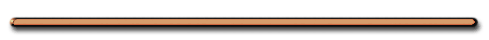HomeIntermolecular bonds between
water molecules are very strong

Video Instruction

showmethephysics.com

D. Finding resultants when angle between forces = 90°

 Ex 1 ) A 5.0 N force pulls an object north while a 12 N force is pulling the object west.   Find the resultant

Scale 1 cm = 1 N???

Two Methods

Method One

Graphical Method

(Draw It!!)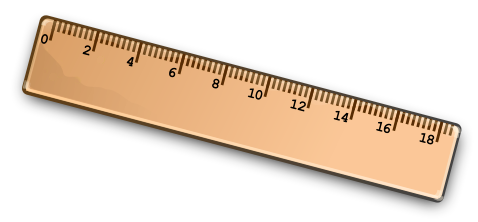1. Draw vectors to scale (1 N = 1 cm)2. Make a parallelogram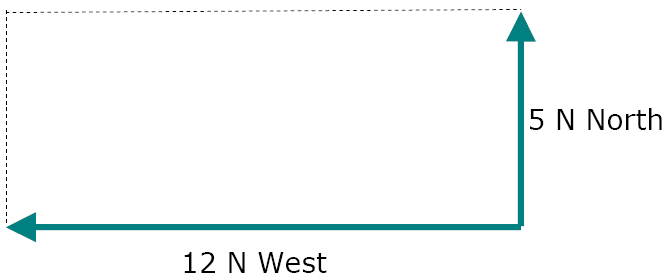Protractor 101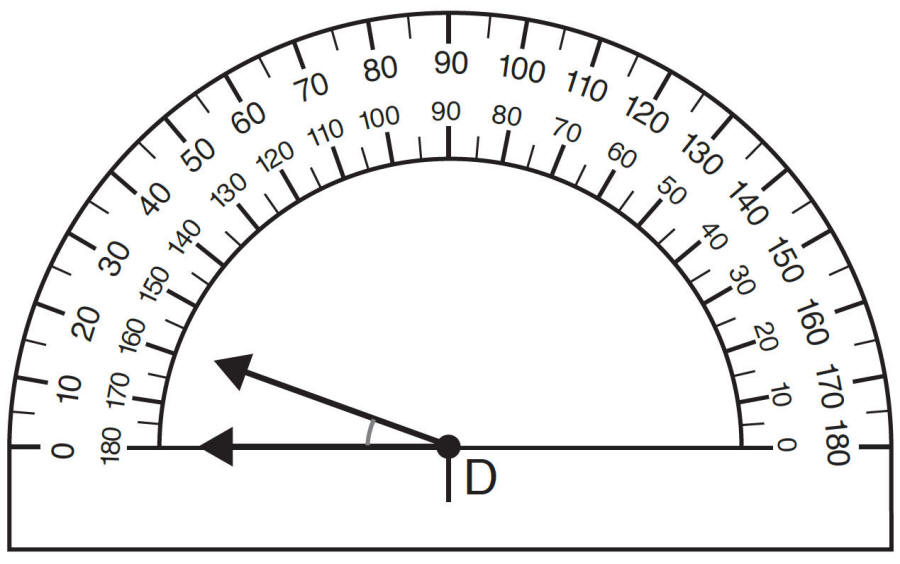1. Use the Acute Angles Only 2. Use a ruler to get accurate measurements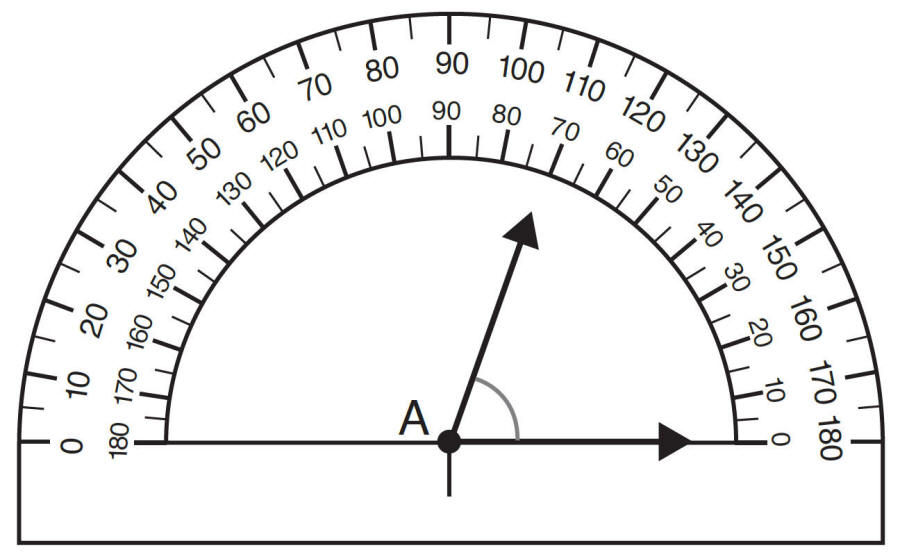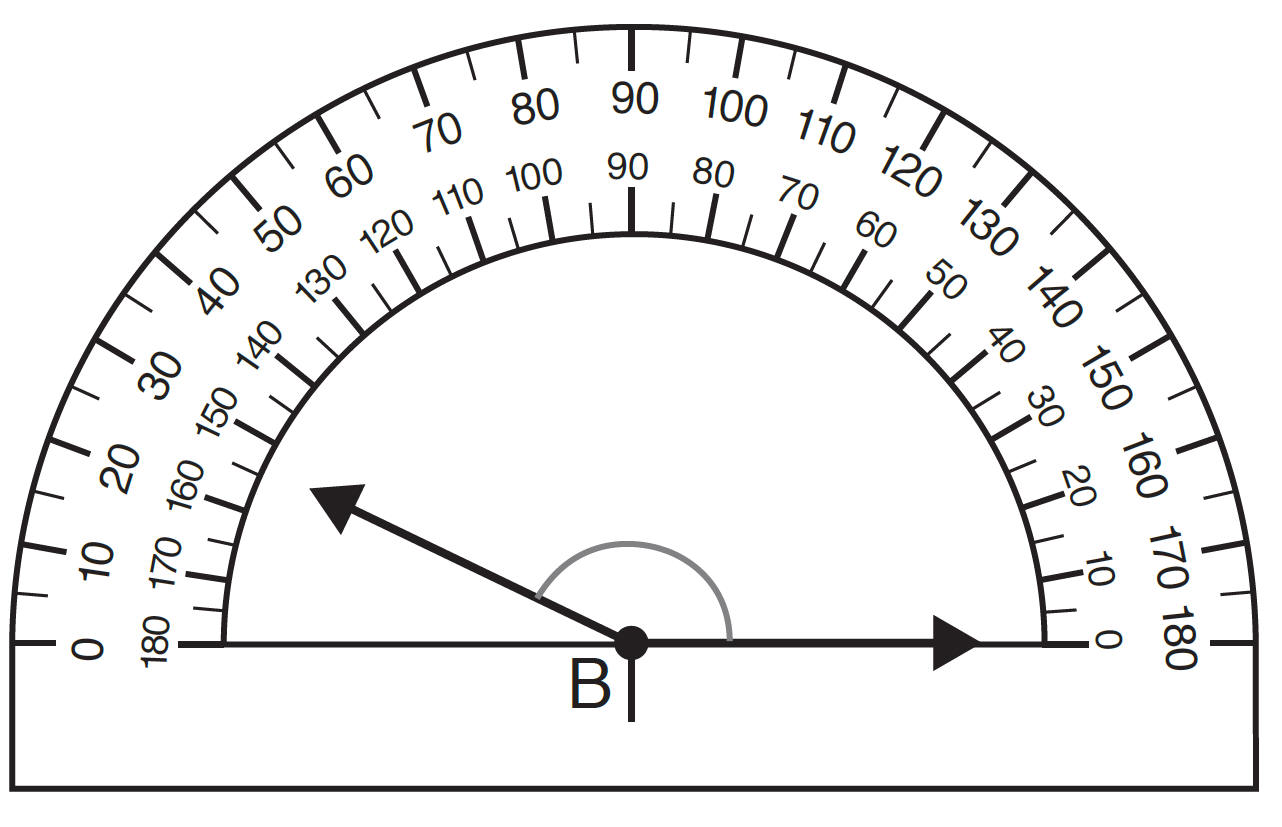3. Draw diagonal (resultant)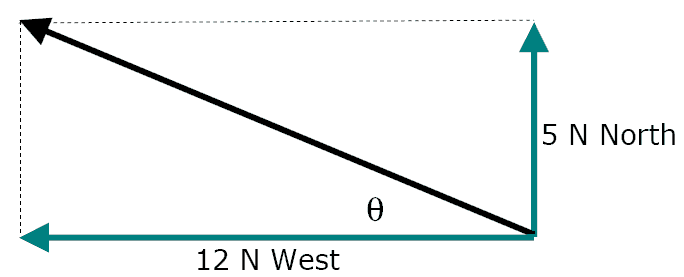4. Find magnitude & direction of vector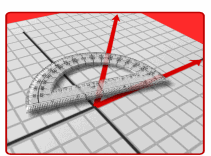Parallelogram Method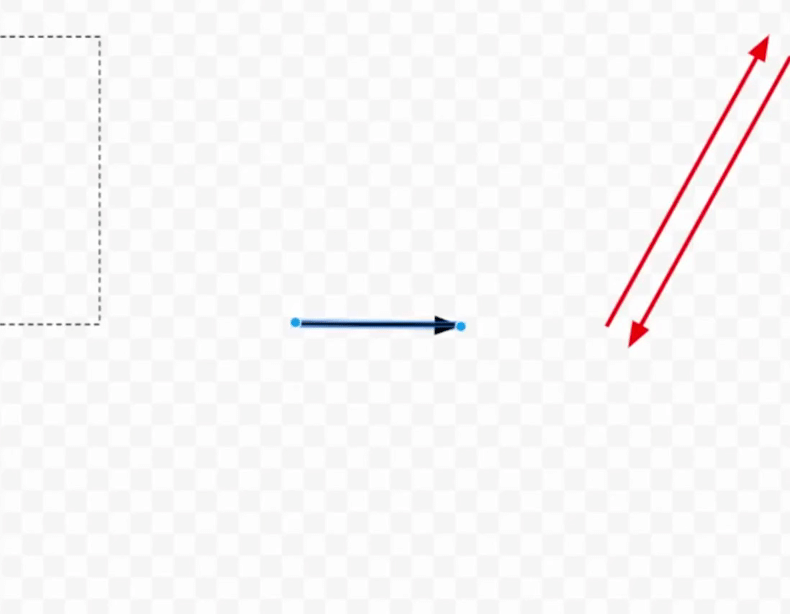Method Two

- Trig. and Pythagorean
(More accurate)

1. Use a2 + b2 = c2 (To find magnitude)

5.0 N2  + 12 N2  = R2TanӨ = 5.0/12

Ө = ?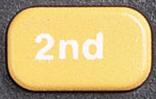Tan (5/12)

Inverse Tangent (5 /12)13 N  22.6 ° N of WName that Direction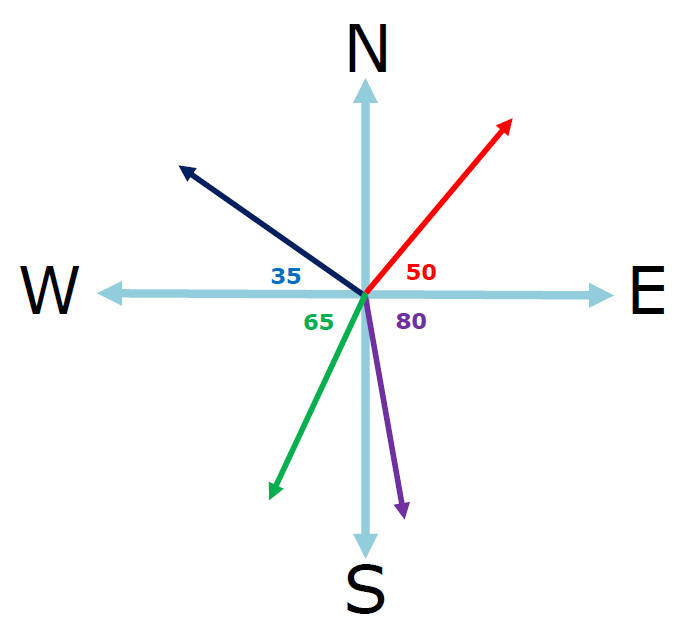50 degrees N of E, 35 degrees N of W

65 degrees S of W, 80 degrees S of E

Video Instruction

showmethephysics.com

E. Equilibrant - Balancing ForceShow Me The Physics

Video Instruction

showmethephysics.com

The Equilibrant is always equal in magnitude and opposite in direction to the resultant

[ Flash ]

If…

Resultant  = 5 N 53° N of W

Then…

Equilibrant  =

5 N 53° S of E

Video Instruction

showmethephysics.com

Ex 2) Concurrent forces (acting together) of 10. nt east and 10. nt south act on an object.

Resultant & Equilibrant using
Trig. & Pythagorean Theorem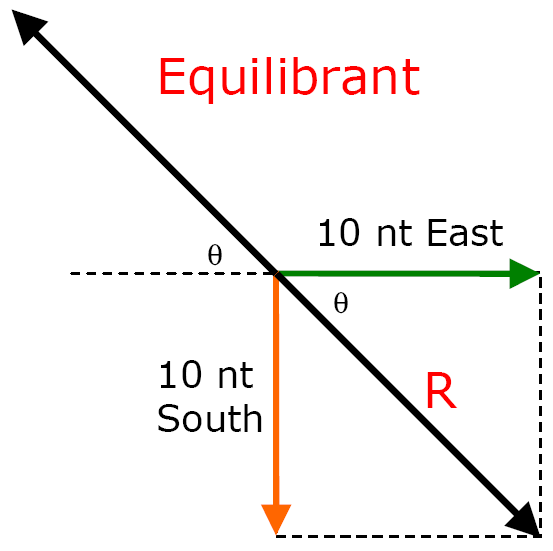1.  10 N2 + 10 N2 = c2

c = 14 nt

2.  R = 14 nt 45° S of E

Equilibrant  = 14 nt  45° N of W

Let's go to the Videotape!